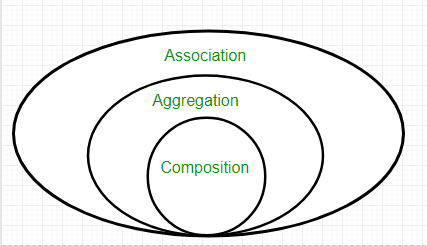# Composition in Java

The composition is a design technique in java to implement a has-a relationship. Java Inheritance is used for code reuse purposes and the same we can do by using composition. The composition is achieved by using an instance variable that refers to other objects. If an object contains the other object and the contained object cannot exist without the existence of that object, then it is called composition. In more specific words composition is a way of describing reference between two or more classes using instance variable and an instance should be created before it is used.The benefits of using Composition is as follows:

1. Composition allows the reuse of code.
2. Java doesn’t support multiple inheritances but by using composition we can achieve it.
3. Composition offers better test-ability of a class.
4. By using composition, we are flexible enough to replace the implementation of a composed class with a better and improved version.
5. By using composition, we can also change the member objects at run time, to dynamically change the behaviour of your program.

Do remember the certain key points of composition in java which are as follows:

• It represents a has-a relationship.
• In composition, both entities are dependent on each other.
• When there is a composition between two entities, the composed object cannot exist without the other entity. For example, A library can have no. of books on the same or different subjects. So, If the Library gets destroyed then All books within that particular library will be destroyed. This is because books can not exist without a library.
• The composition is achieved by using an instance variable that refers to other objects.
• We have to favour Composition over Inheritance.

Now let us finally refer to the below image in order to get a faint hint about the aggregation and to better understand how the composition works, let us take an example of the real-time library system.

Real-life Example: Library system

Let’s understand the composition in Java with the example of books and library. In this example, we create a class Book that contains data members like author, and title and create another class Library that has a reference to refer to the list of books. A library can have no. of books on the same or different subjects. So, If the Library gets destroyed then All books within that particular library will be destroyed. i.e., books can not exist without a library. The relationship between the library and books is composition.

Implementation:

Example

## Java

 `// Java program to Illustrate Concept of Composition`   `// Importing required classes` `import` `java.io.*;` `import` `java.util.*;`   `// Class 1` `// Helper class` `// Book class` `class` `Book {`   `    ``// Member variables of this class` `    ``public` `String title;` `    ``public` `String author;`   `    ``// Constructor of this class` `    ``Book(String title, String author)` `    ``{`   `        ``// This keyword refers top current instance` `        ``this``.title = title;` `        ``this``.author = author;` `    ``}` `}`   `// Class 2` `// Helper class` `// Library class contains list of books.` `class` `Library {`   `    ``// Reference to refer to list of books.` `    ``private` `final` `List books;`   `    ``// Constructor of this class` `    ``Library(List books)` `    ``{`   `        ``// This keyword refers to current instance itself` `        ``this``.books = books;` `    ``}`   `    ``// Method of this class` `    ``// Getting the list of books` `    ``public` `List getListOfBooksInLibrary()` `    ``{` `        ``return` `books;` `    ``}` `}`   `// Class 3` `// Main class` `class` `GFG {`   `    ``// Main driver method` `    ``public` `static` `void` `main(String[] args)` `    ``{`   `        ``// Creating the objects of class 1 (Book class)` `        ``// inside main() method` `        ``Book b1` `            ``= ``new` `Book(``"EffectiveJ Java"``, ``"Joshua Bloch"``);` `        ``Book b2` `            ``= ``new` `Book(``"Thinking in Java"``, ``"Bruce Eckel"``);` `        ``Book b3 = ``new` `Book(``"Java: The Complete Reference"``,` `                           ``"Herbert Schildt"``);`   `        ``// Creating the list which contains the` `        ``// no. of books.` `        ``List book = ``new` `ArrayList();`   `        ``// Adding books to List object` `        ``// using standard add() method` `        ``book.add(b1);` `        ``book.add(b2);` `        ``book.add(b3);`   `        ``// Creating an object of class 2` `        ``Library library = ``new` `Library(book);`   `        ``// Calling method of class 2 and storing list of` `        ``// books in List Here List is declared of type` `        ``// Books(user-defined)` `        ``List books` `            ``= library.getListOfBooksInLibrary();`   `        ``// Iterating over for each loop` `        ``for` `(Book bk : books) {`   `            ``// Print and display the title and author of` `            ``// books inside List object` `            ``System.out.println(``"Title : "` `+ bk.title` `                               ``+ ``" and "` `                               ``+ ``" Author : "` `+ bk.author);` `        ``}` `    ``}` `}`

Output

```Title : EffectiveJ Java and  Author : Joshua Bloch
Title : Thinking in Java and  Author : Bruce Eckel
Title : Java: The Complete Reference and  Author : Herbert Schildt```

Whether you're preparing for your first job interview or aiming to upskill in this ever-evolving tech landscape, GeeksforGeeks Courses are your key to success. We provide top-quality content at affordable prices, all geared towards accelerating your growth in a time-bound manner. Join the millions we've already empowered, and we're here to do the same for you. Don't miss out - check it out now!

Previous
Next